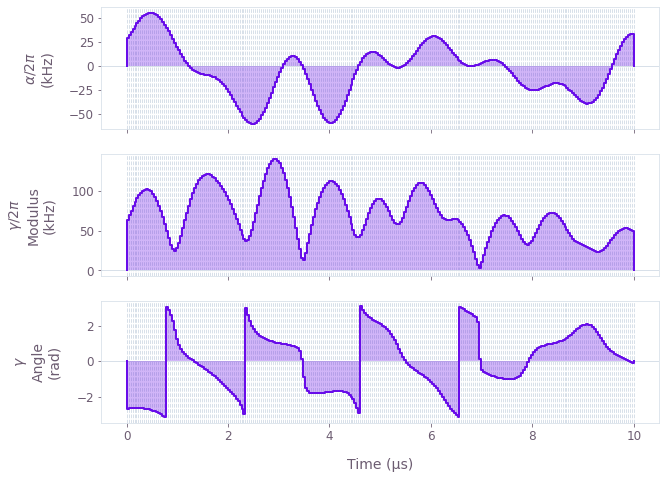•Boulder Opal
• User guides
• Optimize controls in arbitrary quantum systems using graphs

# How to optimize controls in arbitrary quantum systems using graphs

Highly-configurable non-linear optimization framework for quantum control

Boulder Opal provides a highly-flexible optimization engine for general-purpose gradient-based optimization. It can be directly applied to model-based control optimization for arbitrary-dimensional quantum systems.

The optimization engine from Boulder Opal allows the user to express their system Hamiltonians as almost-arbitrary functions of the controllable parameters. The underlying structure of this map is a graph, which defines the cost function and can be efficiently evaluated and differentiated. The resulting optimized controls thus achieve the desired objectives within the constraints imposed by the user-defined Hamiltonian structure.

The example in this user guide illustrates how to optimize multiple controls under different constraints in a single system. For a step-by-step description of how to create a robust optimization with multiple controls, see our tutorial Design robust single-qubit gates using computational graphs.

## Summary workflow

### 1. Define the computational graph

The Boulder Opal optimization engine expresses all optimization problems as data flow graphs, which you can create with the qctrl.create_graph function. The methods of the graph object allow you to represent the mathematical structure of the problem that you want to solve.

For an optimization, a typical workflow is to:

• Create "signals", or scalar-valued functions of time, which typically represent control pulses.
• Create "operators", or matrix-valued functions of time, by modulating constant operators by signals. These typically represent terms of a Hamiltonian.
• Combine the operators into a single Hamiltonian operator.
• Calculate the optimization cost function (typically an infidelity) from the Hamiltonian.

### 2. Execute graph-based optimization

You can calculate an optimization from an input graph using the qctrl.functions.calculate_optimization function. Provide the name of the node of the graph that represents the cost, and this function will return the optimized value of the output nodes that you requested.

## Example: Optimal control of a single qubit

This example shows how to optimize a Hamiltonian with multiple controls. Specifically, consider a single-qubit system represented by the following Hamiltonian:

\begin{align} H(t) &= \frac{\nu}{2} \sigma_{z} + \frac{1}{2}\left[\gamma(t)\sigma_{-} + \gamma^*(t)\sigma_{+}\right] + \frac{\alpha(t)}{2} \sigma_{z} , \end{align}

where $\nu$ is the qubit detuning, $\gamma(t)$ and $\alpha(t)$ are, respectively, complex and real time-dependent pulses, $\sigma_{\pm}$ are the qubit ladder operators, and $\sigma_{z}$ is the Pauli-Z operator.

The functions of time $\gamma(t)$ and $\alpha(t)$ are not predetermined, and instead are optimized by Boulder Opal in order to achieve the target operation, which in this case is a Y-gate.

import numpy as np
from qctrlvisualizer import plot_controls

from qctrl import Qctrl

# Start a Boulder Opal session.
qctrl = Qctrl()
# Define physical constants.
nu = 2 * np.pi * 5e5  # rad/s
gamma_max = 2 * np.pi * 3e5  # rad/s
alpha_max = 2 * np.pi * 1e5  # rad/s
cutoff_frequency = 5e6  # Hz
segment_count = 50
duration = 10e-6  # s

# Create the graph describing the system.
graph = qctrl.create_graph()

# Create the time-independent detuning term.
detuning = nu * graph.pauli_matrix("Z") / 2

# Create a optimizable complex-valued piecewise-constant (PWC) signal.
rough_gamma = graph.utils.complex_optimizable_pwc_signal(
segment_count=segment_count, maximum=gamma_max, duration=duration
)
# Smooth the signal.
gamma = graph.utils.filter_and_resample_pwc(
pwc=rough_gamma,
segment_count=256,
cutoff_frequency=cutoff_frequency,
name=r"$\gamma$",
)

# Create a PWC operator representing the drive term.
drive = graph.hermitian_part(gamma * graph.pauli_matrix("M"))

# Create an optimizable real-valued PWC signal.
rough_alpha = graph.utils.real_optimizable_pwc_signal(
segment_count=segment_count,
minimum=-alpha_max,
maximum=alpha_max,
duration=duration,
)
# Smooth the signal.
alpha = graph.utils.filter_and_resample_pwc(
pwc=rough_alpha,
segment_count=256,
cutoff_frequency=cutoff_frequency,
name=r"$\alpha$",
)
# Create a PWC operator representing the clock shift term.
shift = alpha * graph.pauli_matrix("Z") / 2

# Define the total Hamiltonian and the target operation.
hamiltonian = detuning + drive + shift
target = graph.target(graph.pauli_matrix("Y"))

# Create the infidelity.
infidelity = graph.infidelity_pwc(hamiltonian, target, name="infidelity")

# Run the optimization.
result = qctrl.functions.calculate_optimization(
graph=graph,
cost_node_name="infidelity",
output_node_names=[r"$\alpha$", r"$\gamma$"],
optimization_count=4,
)
print(f"Optimized cost:\t{result.cost:.3e}")

# Plot the optimized controls.
plot_controls(result.output)
Your task calculate_optimization (action_id="1599294") has completed.
Optimized cost:	1.667e-12This notebook was run using the following package versions. It should also be compatible with newer versions of the Q-CTRL Python package.

PackageVersion
Python3.10.8
matplotlib3.6.3
numpy1.24.1
scipy1.10.0
qctrl20.1.1
qctrl-commons17.7.0
boulder-opal-toolkits2.0.0-beta.3
qctrl-visualizer4.4.0